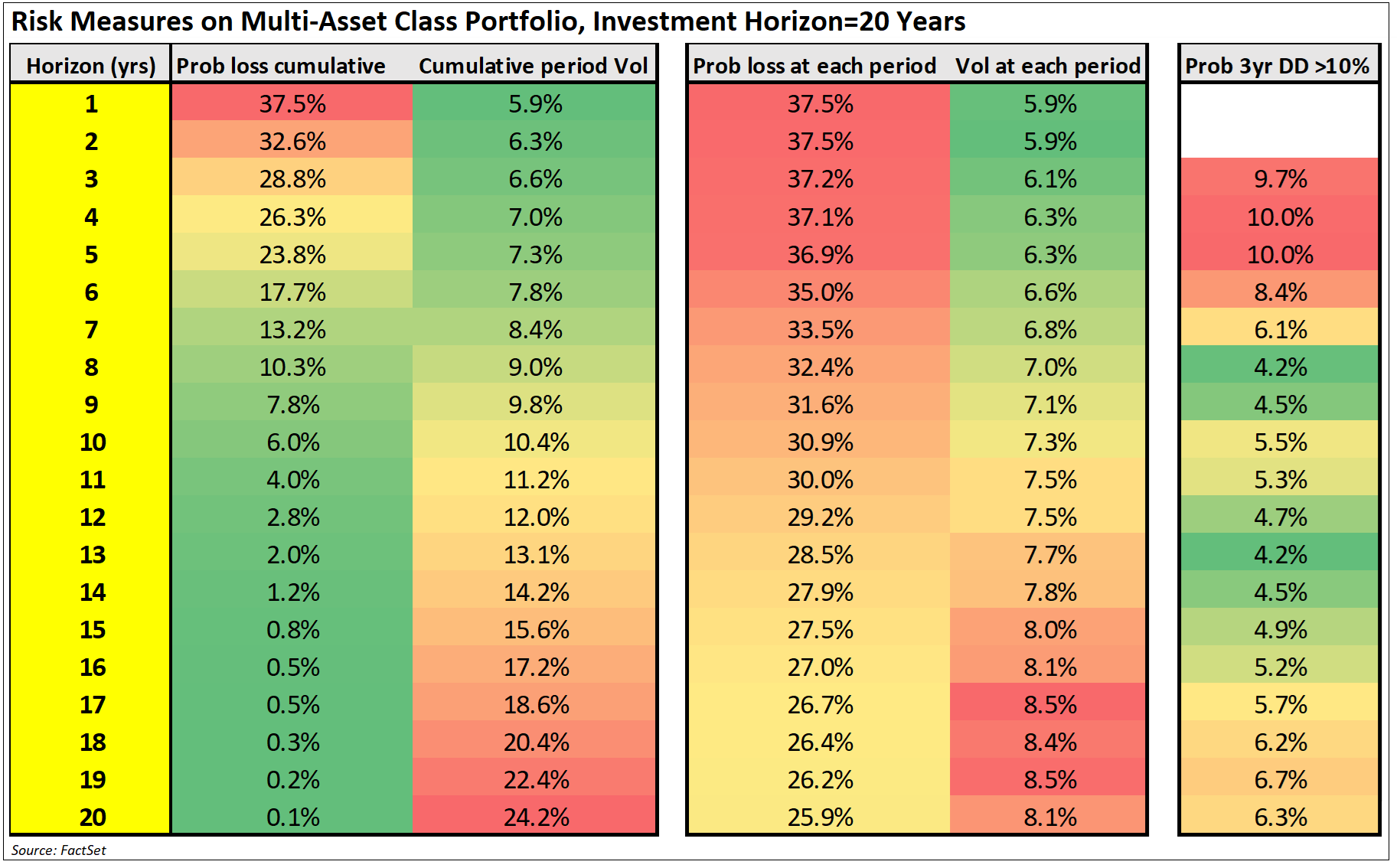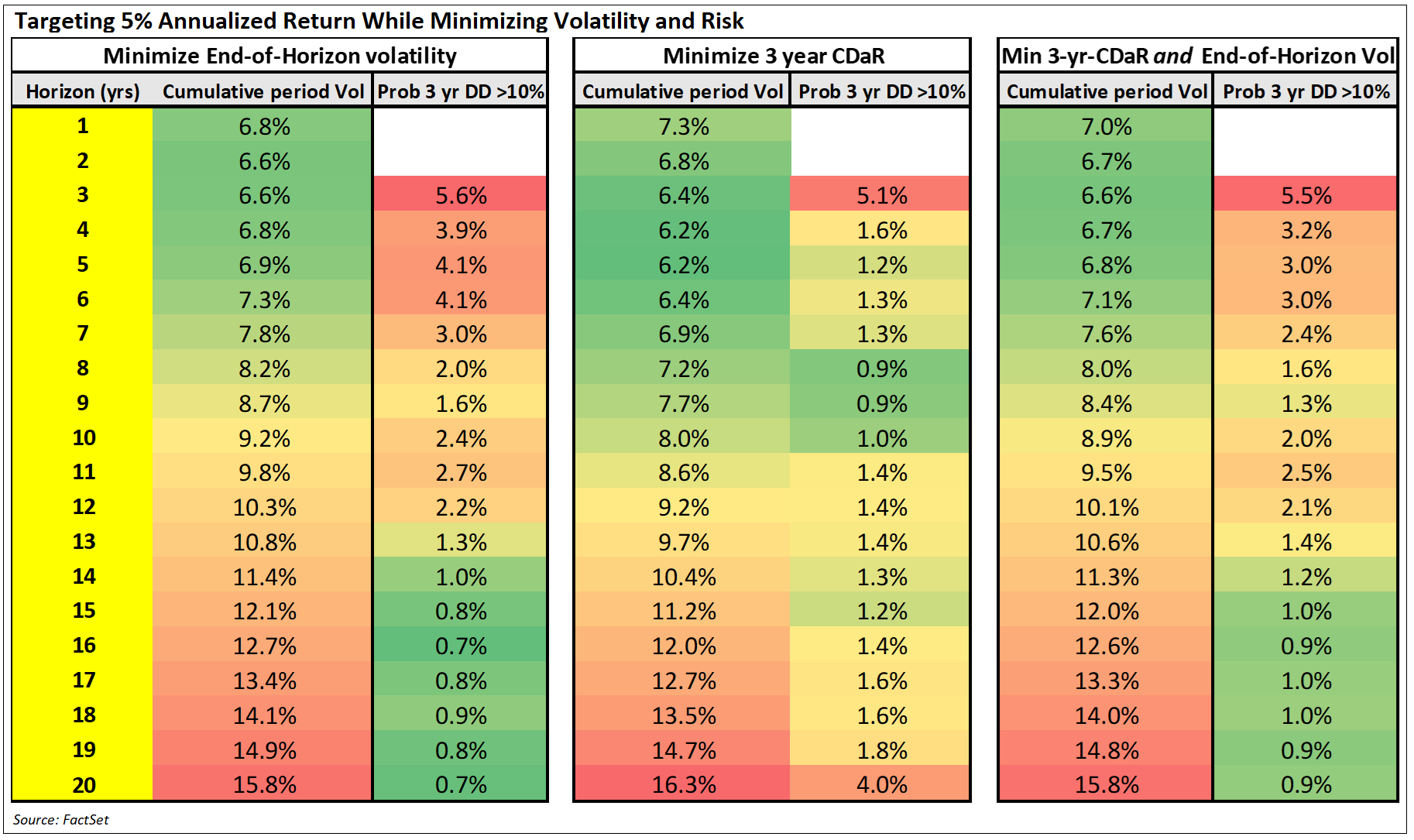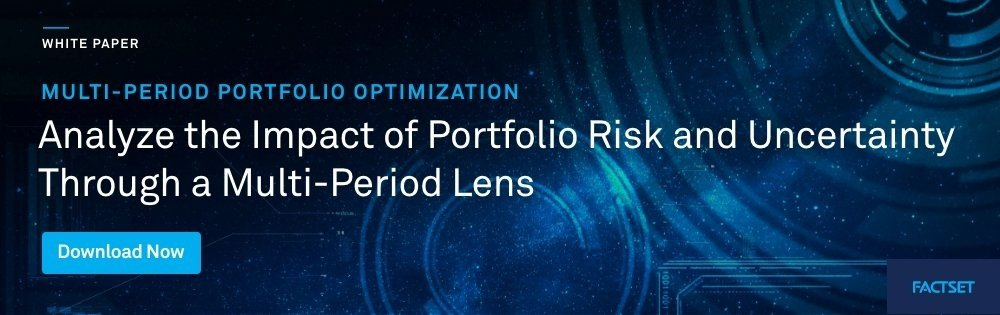# What is the Right Risk Measure for Long-Term Investors?

###### Risk, Performance, and Reporting

By Chen Sui, CFA  |  August 4, 2022

Long-term investors will need to adopt next-generation strategic asset allocation practices to successfully play their part in a rapidly changing world. Truths, practices, and principles traditionally accepted by long-term investors face reduced significance and potential obsolescence. In this series, we examine the key topics and expected changes to how long-term investors will make investment decisions. In this article, we examine how multi-horizon analysis can play a crucial role in managing risk for long-term investors.

Long-term investors frequently analyze forward-looking investment risk with more emphasis on techniques focused on shorter-term horizons (measured in days and weeks) rather than long-term (measured in months and years). The usage of shorter-term ex-ante investment risk models is crucial to assess investment risk and establish controls; the process is well established and investment risk solutions are mature.

It’s time to give long-term investment risk the same focus and further manage risk in a multi-horizon way. After all, long-term investors are all balancing both long-term investment goals and obligations with the need for shorter-term and within-horizon risk monitoring and analysis.

## Calculating Long-Term Investment Risk

Investment risk is the failure to reach investment objectives; long-term investors should be managing risk consistently with long-term objectives.

Often short-term investment risk measures are extended to long-term horizons by calculating an end-of-horizon risk without due care to the investor needs within the investment horizon. This can lead to conclusions that are not appropriate for a given investor.

To illustrate how different risk measures can lead to different conclusions, we calculated risk measures on a multi-asset class portfolio with an investment horizon of 20 years. The portfolio is constructed by targeting an annualized return of 5% while minimizing volatility. Both end-of-horizon and within-horizon risk statistics are calculated using the same set of simulated portfolio values projected out 20 years and assuming a buy-and-hold rebalancing strategy.### Observations

1. Probability of loss cumulative. With the end-of-horizon cumulative probability of loss, when looking at all the simulated portfolio outcomes at year 15, only 0.8% of observations show a negative return after 15 years. The longer the investment horizon, the lower the end-of-horizon probability of loss. This leads to an often-used heuristic: time-diversification, the concept of longer investment horizons reducing the probability of loss.
2. Cumulative period volatility. The end-of-horizon cumulative period volatility (annualized) tells us that the standard deviation of the simulated portfolio outcomes at year 15 is 15.6%, and by year 20, it is 24.2%. This is always increasing with time.
3. Probability of loss at each period. The within-horizon (each year) probability of loss measures the number of simulated returns which are negative during each year. For example, there is a 27.5% chance of suffering a loss in year 15 and that probability gradually decreases the longer the investment horizon.
4. Volatility at each period. The within-horizon (each year) volatility generally increases over time.
5. Probability 3-year drawdown > 10%. This is the within-horizon (rolling three years) probability of suffering a drawdown greater than 10% calculated using annual frequency. A drawdown of 10% may not seem like a large peak-to-trough drawdown; however, a 10% drawdown calculated at an annual frequency is empirically similar to a 20% drawdown calculated using a monthly frequency. There is no clear trend throughout the 20-year investment horizon.

## Are You Using the Right Measure of Risk?

All the metrics in the table above can be considered measures of investment risk and are calculated on the same portfolio and inputs.

So why do they indicate different things? Those metrics can support a conclusion of risk increasing over time, decreasing over time, or increasing and then decreasing. What is the right interpretation of how the risk is changing over time? Surely, they can’t all be right.

The answer is that all the risk measures are “correct” but the right one to use very much depends on the investor, how they experience risk, and how frequently they evaluate the portfolio.

An investor that only cares about minimizing the probability of loss at the end of the investment horizon should look at the end-of-horizon cumulative probability of loss (0.1% after 20 years). Investors that care about within-horizon probability of loss should pay more attention to the probability of loss at each period (ranges between 37.5% to 25.9%). Issues occur where investor expectations are set using a low end-of-horizon probability of loss and is interpreted to mean a low within-horizon probability of loss.

Often investment performance is judged relative to a psychological “anchor” at a recent high point; portfolio losses are then judged against this point. This is a different concept to risk defined using volatility and probability of loss—and a common and often headline-grabbing way to measure investment losses. If the investor experiences risk as drawdown, it makes sense that this should be the risk measure to use.

## The Role of Multi-Horizon Risk

Selecting the right risk measure for a particular investor is useful not just to communicate the risk of not achieving an investment goal; it also has implications on portfolio construction. An investment strategy that focuses on a low probability of loss at the end of the investment horizon is unlikely to be suitable for investors that are alarmed at within-horizon losses.

Long-term investors rarely have the luxury of only paying attention to risk measured at the end-of-horizon. Instead, each investor needs to balance the end-of-horizon risks with shorter-term within-horizon risk measures to ensure that they don’t jeopardize the ability to continue with a particular investment strategy.

Multi-horizon investment risk is focused on managing risk across multiple horizons concurrently to help investors with this balancing act.

## How Can You Apply Multi-Horizon Risk in Portfolio Construction?

Within-horizon risk (e.g., volatility per period and drawdown), measures by definition divide the investment horizon into multiple periods, so it falls within the scope of multi-period analysis. We can use a multi-period portfolio optimizer introduced previously to help investors strike the balance between end-of-horizon and within-horizon risk measures and at the same time, more accurately model real-world behavior (intra-period rebalancing) throughout the investment horizon.

Using the same capital market assumptions (multi-period return inputs) as in our previous article, we compare investment strategies that target an annualized return of 5% while minimizing end-of-horizon volatility, within-horizon drawdown risk (conditional-drawdown-at-risk [CDaR]), and both end-of-horizon volatility and within-horizon drawdown risk.### Observations

1. Minimizing end-of-horizon volatility. This uses a dynamic rebalancing strategy and can be considered the base case. Note that compared with the buy-and-hold approach, the overall volatility is much lower (15.8% vs. 24.2%) since the portfolio is allowed to dynamically rebalance allocations at each period.
2. Minimize 3-year CDaR. When the objective of the portfolio is to minimize CDaR, unsurprisingly drawdown risk is reduced relative to (1), albeit at the expense of end-of-period volatility.
3. Minimize 3-yr CDaR and End-of-Horizon Volatility. For those investors that need to balance both end-of-horizon risk and within-horizon risk, it’s possible to include both objectives into the multi-period optimizer. In this case, the result is a small improvement to the drawdown risk that can be achieved without increasing end-of-horizon volatility.

## Conclusion

Knowing the risk measures that are important to an investor, it is possible to improve the investor experience by constructing a portfolio that manages that particular risk measure.

“If you can measure it, you can manage it.”

Until recently, these risk measures have been dominated by short-term risk and end-of-horizon risk measures. This is missing the within-horizon element of risk, which so frequently derails even the best-laid strategies.

Long-term investors have found within-horizon risk to be tricky to measure and manage since it is a multi-period concept and certain measures like drawdown rely on path-dependent techniques. With the latest forward-looking multi-period simulation and multi-period optimization techniques, investors can create strategies that balance both the end-of-horizon risk and within-horizon risks.

Disclaimer: This blog post is for informational purposes only. The information contained in this blog post is not legal, tax, or investment advice. FactSet does not endorse or recommend any investments and assumes no liability for any consequence relating directly or indirectly to any action or inaction taken based on the information contained in this article.## Chen Sui, CFA

###### Principal Product Manager, Analytics and Trading Solutions

Mr. Chen Sui is a Principal Product Manager for Analytics and Trading Solutions at FactSet. In this role, he is focuses on helping clients adopt long-term investment behavior through the adoption of ideas and practices best suited to long-term investors. He has over 15 years of experience working with analytics clients across the globe and a wide variety of buy-side institutions such as traditional institutional asset managers, asset owners, hedge funds, and wealth management firms. Prior, he spent time in performance, risk, project, and client-servicing teams at Wilshire Associates, JP Morgan, and CQS. Mr. Sui earned a master’s degree in Mathematical Finance from the University of York and is a CFA charterholder.